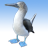QlikView App Dev

Discussion Board for collaboration related to QlikView App Development.

Announcements
QlikWorld 2022, LIVE in Denver CO., May 16-19, 2022. REGISTER NOW TO RECEIVE EARLY BIRD PRICING
cancel
Showing results for
Did you mean:Contributor II

Group condition in Set Analysis

I have a productid , productType

I need to have

sum of sales  when (prodctid = 4 and productType = ("a","b","c","d") ) or (productId = 5 and ProuctType =("a","d","f"))

I am able to make two conditions but not a same single one for the above requirement.

Need Help with this.

5 RepliesMVP

Try union set operator + like in

=Sum( {<productid = {4}, productType = {'a','b','c','d'}> + <productid = {5}, productType = {'a','d'.'f'}>} sales)Contributor II
Author

Thanks, That Helps

now i am confused.

Can we write a compound condition in set analysis like

sum of sales ( compid = 1 , prefferedrep=4, (prodctid = 4 and productType = ("a","b","c","d") ) or (productId = 5 and ProuctType =("a","d","f"), date between Jan 01 to Jan 31)

Sum ({< compid = {'1'}

, prefferdredp = {'4'}

,date = {'>= \$(vYearStartDate) <= \$(vYearEndDate)'}

>}sales ) is the condition so far , now need to add the Sum( {<productid = {4}, productType = {'a','b','c','d'}> + <productid = {5}, productType = {'a','d'.'f'}>} sales) into the existing sum .MVP

May be try this:

Sum ({

<compid = {'1'}, prefferdredp = {'4'}, date = {'>= \$(vYearStartDate) <= \$(vYearEndDate)'}, productid = {4}, productType = {'a','b','c','d'}>

+

<compid = {'1'}, prefferdredp = {'4'}, date = {'>= \$(vYearStartDate) <= \$(vYearEndDate)'}, productid = {5}, productType = {'a','d'.'f'}>}

sales)MVP

Just like selections in list boxes, set analysis using a field selection uses an OR logic within the field selection and AND logic when combining several field selections. If you need another logic, use several set modifier (the part between the angle brackets <...> in combination with set operators.

Sum( {

<compid = {'1'}, prefferdredp = {'4'} ,date = {'>= \$(vYearStartDate) <= \$(vYearEndDate)'},

productid = {4}, productType = {'a','b','c','d'}>

+

<compid = {'1'}, prefferdredp = {'4'},date = {'>= \$(vYearStartDate) <= \$(vYearEndDate)'},

productid = {5}, productType = {'a','d','f'}>

} sales)Contributor II
Author

Got to get this below condition into a straight table expression.

sum (Net_amount)  when

bd_id = 1

and ass.sec_type = 'mf'

and cancelled_ind = 'N'

and proprietary = 0

and trans_type = 'b'

and int_type not in (0, -1, 25, 207, 6, 7, 24, 11, 30, 31, 43, 51, 52, 211)

and cusip not like 'FDN%'

and trade_date between '1/01/2016' and '3/31/2016'

and acct_type not like '%529%'

and net_amt >= \$2000

and Norm_Trans_Cd not in ('0301SDU','0302SDU','0401SUD','0402RDU','4001SDU')

and ((trans_code not in ('20XX  1','050',   '052',  '332300++','3320++', '132101++','10TOAD','0140','002'))   or exchange_ind = 'N')

got to this

<[BD_ID] = {'1'}

,[Description] = {'Independent RIA', 'Advisor Select – Full Wrap', 'Advisor Select – with Trans Chrgs'}

,[trans_type] ={'b'}

,[Int_Type]-={'0','-1','25','207','6','7','24','11','30','31','43','51','52','211'}

,[sec_type]-={'mm', 'ca', '01'}

,[CUSIP] -= {'FDN*'}

,[Acct_Type] -={'*529*'}

,[Cancelled_Ind] = {'N'}

,[Proprietary] = {'0'}

,[Trans_Type] = {'b'}

,[sec_type] = {'mf','MF'}

,[Norm_Trans_Cd] -={'0301SDU','0302SDU','0401SUD','0402RDU','4001SDU'}

,[Trade_Date]={"\$(= '>=' & \$(vYearStartDate) & '<=' & \$(vYearEndDate))"}

,[Net_Amt] ={">=2000"}